# Why Do AI Engineers Need Calculus

Among many fields and branches of mathematics, Calculus plays a significantly important role in Artificial Intelligence. Today, we’ll cover the basics of what Calculus really is and understand why it is integral for AI Engineers.

# Calculus

Calculus is the study of how things change. It constitutes of two branches namely, differential calculus and integral calculus. Differential calculus explains the rate of change, slopes of curves whereas integral calculus talks about the continuous summation of parts i.e. Areas under the curve. Just as summation describes the addition of discrete parts, integral explains the continuous summation of data.

# What can we do with the things we learned in the previous article Algebra for Machine Learning?

Following the Algebra from the last Article Algebra for Machine Learning, we learned about the functions and equations. It has built a foundation upon which calculus can further take over and solve more complex problems that constitute real-world scenarios from our microwaves, cell phones, TV, and automobiles to medicine, economy, and national defense.

Topic Covered in this article,

• Equation of Functions
• Special functions
1. ln(x)
2. cos(x)
3. 1/(x²-1)
4. tan(x)
• Derivates
• Minima and Maxima
• Concave or convex functions in a defined interval (saddle points)
• Integrals

Equation of Functions,

For an equation,

y= ax + b

Here, x and y are directly proportional to each other.

Ie. y/x = Constant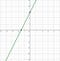Fig: In the above graph, the slope of the line y = 2x +3 is constant.

Linear Function

Linear functions are those functions whose graph represents a straight line. A linear function has the following form. y = f(x) = a + bx. The linear function contains one independent variable and one dependent variable. For the above equation, the independent variable is x and the dependent variable is y.

Linear Regression

Linear regression models the relationship between two different variables by fitting a linear equation to observed data. In linear regression, one of the variables is taken as an explanatory variable, and then another is considered as a dependent variable.

A linear regression line has an equation of the form Y = a + bX, where X is the explanatory variable and Y is the dependent variable. The slope of the line is b, and a is the intercept (the value of y when x = 0).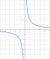Fig: In the graph above, x and y are inversely proportional such that x*y = constant where y = 2/x

# Special functions

Special functions are particular mathematical functions that have more or less established names and notations due to their importance in mathematical analysis, functional analysis, geometry, physics, or other applications. Some of them are listed below,

f(x) = ln(x)

f(x) = x2

f(x) = cos (x)

f(x) = 1 / (x2–1)

f(x) = tan (x)

# Why are we going across these functions?

We are going to come across functions when we need to tune in to minimize our algorithm. We’ll come across different algorithms while exercising Machine Learning Models and it is vital to know how do these functions react and transform it.

# Plotting graph using the function in GeoGebra

GeoGebra is an online web app that provides free tools for learning interactive geometry, algebra, statistics, and calculus application and teaching mathematics and science from primary school to university level.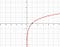Fig: y = ln(x)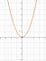Fig: y = x^2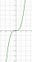Fig: y = x^3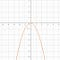Fig: y = -x^2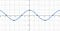Fig: y = cos(x)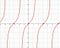Fig: y = tan(x)

# Derivatives

Derivatives describe and explain the change i.e.. the slope of a function. It measures the sensitivity of the change in a particular function i.e.. The output value with respect to the change in the input value.

For an analogy of where Derivatives are directly used in ML, while developing Machine Learning Models for Autonomous Driving Vehicles, without Derivative, Instantaneous Velocity and Acceleration would be impossible to calculate for further processing.Fig: Slope is constant across the line

To Read the full article, check out: https://www.c-sharpcorner.com/article/why-do-ai-engineers-need-calculus2/

Man on a Mission - to create epochal impact

## More from Ojash Shrestha

Man on a Mission - to create epochal impact